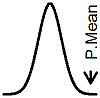P.Mean: Making predictions based on just the correlation (created 2012-03-07). News: Sign up for "The Monthly Mean," the newsletter that dares to call itself average, www.pmean.com/news.Dear Professor Mean, I have a math question. If the correlation, r, between two measurements is 0.1462, and I have one measurement can I calculate the other? I know it probably won't be accurate but can I get a rough approximation?

Knowing r by itself is not enough. If you had more information (in particular the means and standard deviations of the two variables), then you could do it. What you want is to recreate the regression equation. The formula for the slope is

Beta1-hat = Sy*r / Sx

The formula for the intercept is

Beta0-hat = Ybar - Beta1-hat*Xbar

Without the means and standard deviations, the only thing you could do is to state something along the lines of if X is k standard deviations above the mean, then our best estimate of Y is that it is k*0.1462 standard deviations above its mean.This page was written by Steve Simon and is licensed under the Creative Commons Attribution 3.0 United States License. Need more information? I have a page with general help resources. You can also browse for pages similar to this one at Linear Regression.